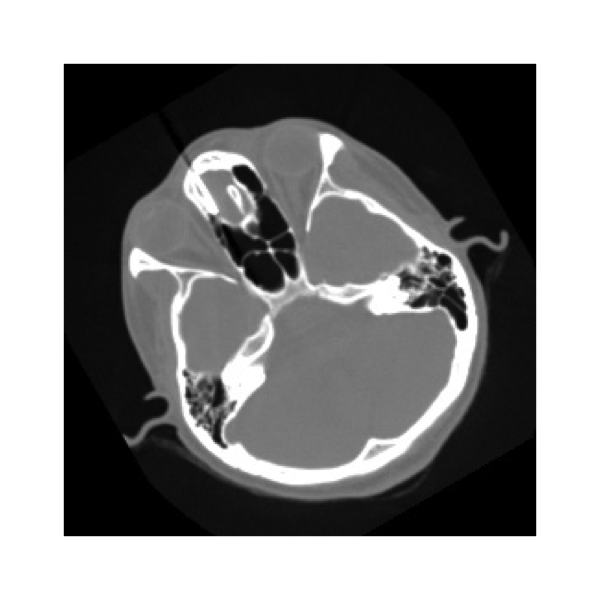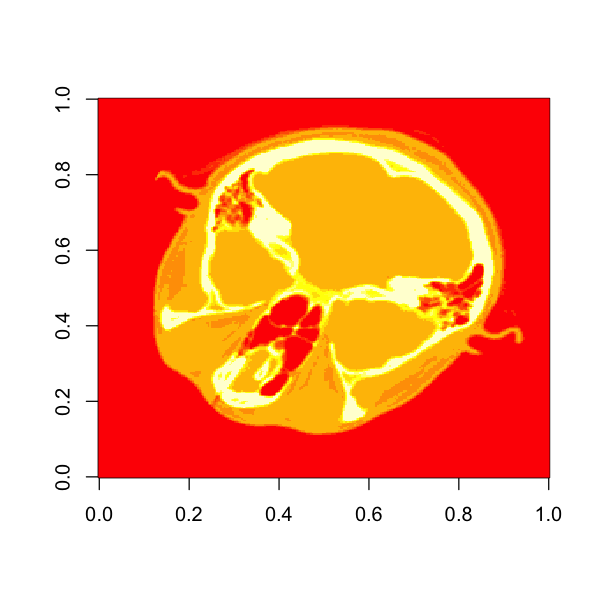# Images and pixels with SimpleITK from R¶

The R notebooks follow the general structure of the python equivalents.

A few comments to get started:

• R will display a variable when you type the name. If the variable is a SimpleITK image then, by default, R/SimpleITK will attempt to display it with ImageJ. This isn't much good for notebooks or knitted documents, so the "show" method has been replaced by a function using internal R graphics. This isn't necessary for interactive sessions.
• The documentation doesn't exist in the R package yet - be prepared to do some detective work.
• C++ enumerated types are wrapped using strings, rather than integers.
• Error messages are can be very cryptic - it helps to get familiar with the R way of querying methods and objects.
• The object oriented style used by swig is one of the less common ones used in R packages.
• C++ vectors are automatically converted to R vectors.

Let's start by creating an image and accessing information about it.

In :
# Load the SimpleITK library
library(SimpleITK)

imA = Image(256, 128, 64, "sitkInt16")
imB = SimpleITK::Image(64, 64, "sitkFloat32")
imC = Image(c(32, 32), "sitkUInt32")
imRGB = Image(c(128,128), "sitkVectorUInt8", 3)


We've created 3 different (empty) images using slightly differing syntax to specify the dimensions. We've also specified the pixel type. The range of available pixel types is:

In :
getAnywhere(".__E___itk__simple__PixelIDValueEnum")

A single object matching ‘.__E___itk__simple__PixelIDValueEnum’ was found
It was found in the following places
namespace:SimpleITK
with value

sitkUnknown          sitkUInt8           sitkInt8         sitkUInt16
-1                  1                  0                  3
sitkInt16         sitkUInt32          sitkInt32         sitkUInt64
2                  5                  4                  7
sitkInt64        sitkFloat32        sitkFloat64 sitkComplexFloat32
6                  8                  9                 10
sitkComplexFloat64    sitkVectorUInt8     sitkVectorInt8   sitkVectorUInt16
11                 13                 12                 15
sitkVectorInt16   sitkVectorUInt32    sitkVectorInt32   sitkVectorUInt64
14                 17                 16                 19
sitkVectorInt64  sitkVectorFloat32  sitkVectorFloat64     sitkLabelUInt8
18                 20                 21                 22
sitkLabelUInt16    sitkLabelUInt32    sitkLabelUInt64
23                 24                 25 

It isn't usually necessary to access the enumeration classes directly, but it can be handy to know how when debugging.

Lets start querying the images we've created. The $ operator is used to access object methods: In : imA$GetSize()
imC$GetSpacing()  1. 256 2. 128 3. 64 1. 1 2. 1 We can change the spacing (voxel size): In : imC$SetSpacing(c(2, 0.25))
imC$GetSpacing()  1. 2 2. 0.25 There are shortcuts for accessing a single dimension: In : imC$GetWidth()
imA$GetHeight() imA$GetDepth()
imC$GetDepth()  32 128 64 0 The depth refers to the z dimension. A colour or vector image has an additional "dimension": In : imRGB$GetNumberOfComponentsPerPixel()

3

The list of available methods can be retrieved using the following. There are many functions for accessing pixels in a type dependent way, which don't need to be accessed directly.

In :
getMethod('$', class(imA))  An object of class “signature” An object of class “signature”  new("MethodDefinition", .Data = function (x, name) { accessorFuns = list(Equal = Image_Equal, GetITKBase = Image_GetITKBase, GetPixelID = Image_GetPixelID, GetPixelIDValue = Image_GetPixelIDValue, GetPixelIDTypeAsString = Image_GetPixelIDTypeAsString, GetDimension = Image_GetDimension, GetNumberOfComponentsPerPixel = Image_GetNumberOfComponentsPerPixel, GetNumberOfPixels = Image_GetNumberOfPixels, GetOrigin = Image_GetOrigin, SetOrigin = Image_SetOrigin, GetSpacing = Image_GetSpacing, SetSpacing = Image_SetSpacing, GetDirection = Image_GetDirection, SetDirection = Image_SetDirection, TransformIndexToPhysicalPoint = Image_TransformIndexToPhysicalPoint, TransformPhysicalPointToIndex = Image_TransformPhysicalPointToIndex, TransformPhysicalPointToContinuousIndex = Image_TransformPhysicalPointToContinuousIndex, TransformContinuousIndexToPhysicalPoint = Image_TransformContinuousIndexToPhysicalPoint, GetSize = Image_GetSize, GetWidth = Image_GetWidth, GetHeight = Image_GetHeight, GetDepth = Image_GetDepth, CopyInformation = Image_CopyInformation, GetMetaDataKeys = Image_GetMetaDataKeys, HasMetaDataKey = Image_HasMetaDataKey, GetMetaData = Image_GetMetaData, SetMetaData = Image_SetMetaData, EraseMetaData = Image_EraseMetaData, ToString = Image_ToString, GetPixelAsInt8 = Image_GetPixelAsInt8, GetPixelAsUInt8 = Image_GetPixelAsUInt8, GetPixelAsInt16 = Image_GetPixelAsInt16, GetPixelAsUInt16 = Image_GetPixelAsUInt16, GetPixelAsInt32 = Image_GetPixelAsInt32, GetPixelAsUInt32 = Image_GetPixelAsUInt32, GetPixelAsInt64 = Image_GetPixelAsInt64, GetPixelAsUInt64 = Image_GetPixelAsUInt64, GetPixelAsFloat = Image_GetPixelAsFloat, GetPixelAsDouble = Image_GetPixelAsDouble, GetPixelAsVectorInt8 = Image_GetPixelAsVectorInt8, GetPixelAsVectorUInt8 = Image_GetPixelAsVectorUInt8, GetPixelAsVectorInt16 = Image_GetPixelAsVectorInt16, GetPixelAsVectorUInt16 = Image_GetPixelAsVectorUInt16, GetPixelAsVectorInt32 = Image_GetPixelAsVectorInt32, GetPixelAsVectorUInt32 = Image_GetPixelAsVectorUInt32, GetPixelAsVectorInt64 = Image_GetPixelAsVectorInt64, GetPixelAsVectorUInt64 = Image_GetPixelAsVectorUInt64, GetPixelAsVectorFloat32 = Image_GetPixelAsVectorFloat32, GetPixelAsVectorFloat64 = Image_GetPixelAsVectorFloat64, GetPixelAsComplexFloat32 = Image_GetPixelAsComplexFloat32, GetPixelAsComplexFloat64 = Image_GetPixelAsComplexFloat64, SetPixelAsInt8 = Image_SetPixelAsInt8, SetPixelAsUInt8 = Image_SetPixelAsUInt8, SetPixelAsInt16 = Image_SetPixelAsInt16, SetPixelAsUInt16 = Image_SetPixelAsUInt16, SetPixelAsInt32 = Image_SetPixelAsInt32, SetPixelAsUInt32 = Image_SetPixelAsUInt32, SetPixelAsInt64 = Image_SetPixelAsInt64, SetPixelAsUInt64 = Image_SetPixelAsUInt64, SetPixelAsFloat = Image_SetPixelAsFloat, SetPixelAsDouble = Image_SetPixelAsDouble, SetPixelAsVectorInt8 = Image_SetPixelAsVectorInt8, SetPixelAsVectorUInt8 = Image_SetPixelAsVectorUInt8, SetPixelAsVectorInt16 = Image_SetPixelAsVectorInt16, SetPixelAsVectorUInt16 = Image_SetPixelAsVectorUInt16, SetPixelAsVectorInt32 = Image_SetPixelAsVectorInt32, SetPixelAsVectorUInt32 = Image_SetPixelAsVectorUInt32, SetPixelAsVectorInt64 = Image_SetPixelAsVectorInt64, SetPixelAsVectorUInt64 = Image_SetPixelAsVectorUInt64, SetPixelAsVectorFloat32 = Image_SetPixelAsVectorFloat32, SetPixelAsVectorFloat64 = Image_SetPixelAsVectorFloat64, SetPixelAsComplexFloat32 = Image_SetPixelAsComplexFloat32, SetPixelAsComplexFloat64 = Image_SetPixelAsComplexFloat64, MakeUnique = Image_MakeUnique, SetPixel = Image_SetPixel, GetPixel = Image_GetPixel) idx = pmatch(name, names(accessorFuns)) if (is.na(idx)) return(callNextMethod(x, name)) f = accessorFuns[[idx]] function(...) { f(x, ...) } }, target = new("signature", .Data = "_p_itk__simple__Image", names = "x", package = "SimpleITK"), defined = new("signature", .Data = "_p_itk__simple__Image", names = "x", package = "SimpleITK"), generic = structure("$", package = "base"))

## Array notation for images¶

R, like python and MATLAB, has a flexible notation for accessing elements of arrays. SimpleITK adopts the same notation for accessing pixels (or sub images, which will be covered in a subsequent notebook). First, lets get set up so that we can display an image in the notebook.

In :
# override show function
# The example below will respect image voxel sizes
## This is a hack to allow global setting of the displayed image width
Dwidth <- grid::unit(10, "cm")

setMethod('show', '_p_itk__simple__Image',
function(object)
{
a <- t(as.array(object))
rg <- range(a)
A <- (a-rg)/(rg-rg)
dd <- dim(a)
sp <- object$GetSpacing() sz <- object$GetSize()
worlddim <- sp*sz
worlddim <- worlddim/worlddim
W <- Dwidth
H <- Dwidth * worlddim
grid::grid.raster(A,default.units="mm", width=W, height=H)
}

)
options(repr.plot.height = 5, repr.plot.width=5)


Typing the name of an image variable will now result in the image being displayed using an R graphics device. Lets load up an image from the package and test:

Now we source the code to allow us to fetch images used in examples. This code will download the images if they aren't already on the system.

In :
source("downloaddata.R")

Loading required package: rPython


In :
cthead <- ReadImage(fetch_data("cthead1.png"))Now we will check the value of a single pixel:

In :
cthead[146, 45]

255

## Pixel access order and conversion to arrays¶

The rule for index ordering in R/SimpleITK is most rapidly changing index first. For images this is x (horizontal), then y (vertical), then z. For R arrays this is row, then column. The conversion between images and arrays maintains the ordering of speed of index change, so the most rapidly changing index is always the first element:

In :
imA\$GetSize()

imA.arr <- as.array(imA)
dim(imA.arr)

1. 256
2. 128
3. 64
1. 256
2. 128
3. 64

The array and image dimensions are the same, however if they are displayed they are likely to appear different as R interprets the first index as rows. Also note that traditional graphing tools are likely to interpret vertical direction differently: For example, lets plot the cthead image - the result is flipped vertically, because the graph origin is bottom left, and rows and columns are swapped. The show method we use in this notebook has a transpose operation to reverse the row/column swap.

In :
image(as.array(cthead))In [ ]: